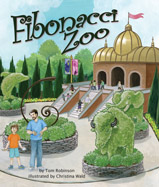Alignment to Standards for VA1 MA-1.21 recognize, describe, extend, and create a wide variety of patterns, including rhythmic, color, shape, and numerical. Patterns will include both growing and repeating patterns. Concrete materials and calculators will be used by students.
1 MA-1.9 create and solve story and picture problems involving one-step solutions, using basic addition and subtraction facts.
2 MA-2.25 identify,create, and extend a wide variety of patterns, using numbers concrete objects and pictures.
2 MA-2.26 solve problems by completing a numerical sentence involving the basic facts for addition and subtraction. Examples include: 3 + __ = 7, or 9 _ __ = 2. Students willcreate story problems, using the numerical sentences.
3 MA-3.24 recognize and describe a variety of patterns formed using concrete objects, numbers, tables, and pictures, and extend the pattern, using the same or different forms (concrete objects, numbers, tables, and pictures).
3 MA-3.25a) investigate andcreate patterns involving numbers, operations (addition and multiplication), and relations that model the identity and commutative properties for addition and multiplication; and
K MA-K.18 identify, describe, and extend a repeating relationship (pattern) found in common objects, sounds, and movements.
K MA-K.2a) tell how many are in the set by counting the number of items orally;
K MA-K.2b) select the corresponding numeral from a given set; and
K MA-K1 The student, given two sets containing 10 or fewer concrete items, will identify and describe one set as having more, fewer, or the same number of members as the other set, using the concept of one-to-one correspondence.

Back to Standards Page